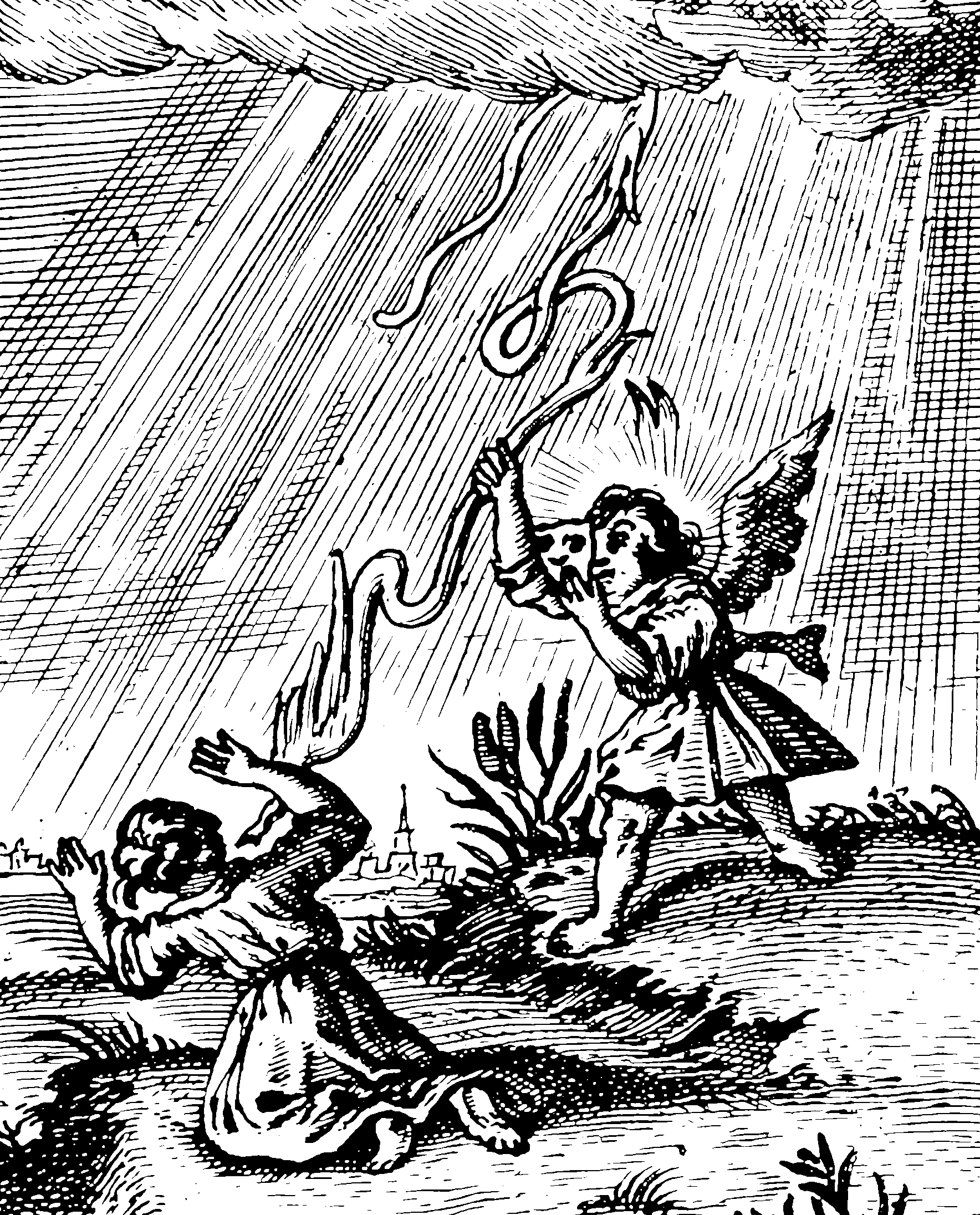# Feedback system identification, not necessarily linear

## Learning dynamics from dataThe order in which this is presented right now makes no sense.

If I have a system whose future evolution is important to predict, why not try to infer a plausible model instead of a convenient linear one?

To reconstruct the unobserved state, as opposed to the parameters of the process acting upon the state, we do state filtering. There can be interplay between these steps, if we are doing simulation-based online parameter inference, as in recursive estimation (what is the division between this and that?) Or: we might decide the state is unimportant and attempt to estimate the evolution only of the observations. That is the Koopman operator trick.

A compact overview is inserted incidentally in Cosma’s review of Fan and Yao (2003) wherein he also recommends .

There are many methods. From an engineering/control perspective, we have , generalises the process for linear time series. to a sparse regression version via Indirect inference, or recursive hierarchical generalised linear models, which is an obvious way to generalise linear systems in the same way GLM generalizes linear models. Kitagawa and Gersch (1996) is popular in a Bayes context.

Hefny, Downey, and Gordon (2015):

We address […] these problems with a new view of predictive state methods for dynamical system learning. In this view, a dynamical system learning problem is reduced to a sequence of supervised learning problems. So, we can directly apply the rich literature on supervised learning methods to incorporate many types of prior knowledge about problem structure. We give a general convergence rate analysis that allows a high degree of flexibility in designing estimators. And finally, implementing a new estimator becomes as simple as rearranging our data and calling the appropriate supervised learning subroutines.

[…] More specifically, our contribution is to show that we can use much-more- general supervised learning algorithms in place of linear regression, and still get a meaningful theoretical analysis. In more detail:

• we point out that we can equally well use any well-behaved supervised learning algorithm in place of linear regression in the first stage of instrumental-variable regression;

• for the second stage of instrumental-variable regression, we generalize ordinary linear regression to its RKHS counterpart;

• we analyze the resulting combination, and show that we get convergence to the correct answer, with a rate that depends on how quickly the individual supervised learners converge

State filters are cool for estimating time-varying hidden states given known fixed system parameters. How about learning those parameters of the model generating your states? Classic ways that you can do this in dynamical systems include basic linear system identification, and general system identification. But can you identify the fixed parameters (not just hidden states) with a state filter?

Yes. This is called recursive estimation.

### Basic Construction

There are a few variations. We start with the basic continuous time state space model.

Here we have an unobserved Markov state process $$x(t)$$ on $$\mathcal{X}$$ and an observation process $$y(t)$$ on $$\mathcal{Y}$$. For now they will be assumed to be finite dimensional vectors over $$\mathbb{R}.$$ They will additionally depend upon a vector of parameters $$\theta$$ We observe the process at discrete times $$t(1:T)=(t_1, t_2,\dots, t_T),$$ and we write the observations $$y(1:T)=(y(t_1), y(t_2),\dots, y(1_T)).$$

We presume our processes are completely specified by the following conditional densities (which might not have closed-form expression)

The transition density

$f(x(t_i)|x(t_{i-1}), \theta)$

The observation density…

TBC.

A trick in differentiation which happens to be useful in differentiating likelihood (or other functions) of time evolving systems using automatic differentiation. e.g. Errico (1997).

## Indirect inference

Popular. See indirect inference.

## Incoming

• Corenflos et al. (2021) describe an optimal transport method
• Campbell et al. (2021) describes variational inference that factors out the unknown parameters.
• Gu et al. (2021) unifies neural ODEs with RNNs.

### No comments yet. Why not leave one?

GitHub-flavored Markdown & a sane subset of HTML is supported.